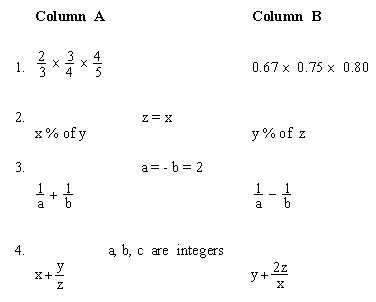Please Support the Monkey! Tell All your Friends and Teachers

PRACTICE TEST 3

Section VII : Quantitative Ability

Time : 30 Minutes                                          30 Questions

Quantitative Comparison Questions 1 - 15

 Directions : In this section you will be given two quantities, one in column A and one in column B. You are to determine a relationship between the two quantities and mark. A) If the quantity in column A is greater than the quantity in column B. B) If the quantity in column B is greater than the quantity in column A. C) If the quantities are equal. D) If the comparison cannot be determined from the information that is given.

 Common Information : Information centered above both columns refers to one or both columns. All numbers used are real numbers. Figures are intended to provide useful positional information, but are not necessarily drawn to scale and should not be used to estimate sizes by measurement. Lines that appear straight can be assumed to be straight.Test 3

Section 1 : Verbal Section

Section 2 : Quantitative Section

Section 3 : Analytical Section

Section 4 : Quantitative Section

Section 5 : Verbal Section

Section 6 : Analytical Section

Section 7 : Quantitative Section

Answer Key To Test 3

Answer Explanation To Test 3
Section 1 : Verbal Section
Section 2 : Quantitative Section
Section 3 : Analytical Section
Section 4 : Quantitative Section
Section 5 : Verbal Section

Section 6 : Analytical Section
Section 7 : Quantitative Section